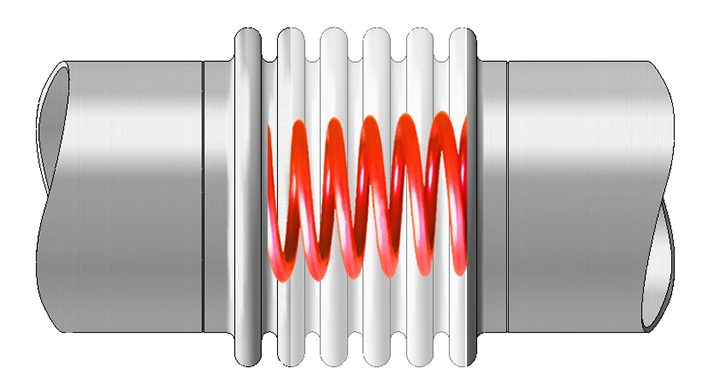# Metal Bellows Spring Rate

The measure of the flexibility of a bellows is known as spring rate and is normally expressed in terms of force required to move the bellows through a specified movement.

Metal bellows spring rate is the force required to compress, extend or angulate the bellows.  Spring rate is expressed as pounds-force per inch (lbf/in.) for compression, extension, and lateral movement, and as pounds force-inch per degree (lbf-in./deg.) for angular movement.

The spring force of metal bellows is calculated as  F = K * X, where:

F is the force imposed on equipment on either end of the bellows
K is the bellows spring rate
X is the specified design movement

The spring force of a bellows is additive to the pressure thrust force unless the pressure thrust force is retained in the metal bellows expansion joint.